Full Text:<1796>

Summary:<610>

CLC number: U491.2

On-line Access: 2013-10-07

Received: 2013-06-06

Revision Accepted: 2013-08-30

Crosschecked: 2013-09-12

Cited: 4

Clicked: 4776

Citations:  Bibtex RefMan EndNote GB/T7714

 Journal of Zhejiang University SCIENCE A 2013 Vol.14 No.10 P.691-704 http://doi.org/10.1631/jzus.A1300208

Analysis of delay variability at isolated signalized intersections*

 Author(s):  Peng Chen1, Huan Liu2, Hong-sheng Qi3, Fu-jian Wang3 Affiliation(s):  1. Department of Civil Engineering, Nagoya University, Furo-cho, Chikusa-ku, Nagoya 464-8601, Japan; more Corresponding email(s):   chenpeng.tjjt@gmail.com Key Words:  Delay variability, Signalized intersection, Cumulative curves, Markov chain Share this article to： More |Next Article >>>

Peng Chen, Huan Liu, Hong-sheng Qi, Fu-jian Wang. Analysis of delay variability at isolated signalized intersections[J]. Journal of Zhejiang University Science A, 2013, 14(10): 691-704.

@article{title="Analysis of delay variability at isolated signalized intersections",
author="Peng Chen, Huan Liu, Hong-sheng Qi, Fu-jian Wang",
journal="Journal of Zhejiang University Science A",
volume="14",
number="10",
pages="691-704",
year="2013",
publisher="Zhejiang University Press & Springer",
doi="10.1631/jzus.A1300208"
}

%0 Journal Article
%T Analysis of delay variability at isolated signalized intersections
%A Peng Chen
%A Huan Liu
%A Hong-sheng Qi
%A Fu-jian Wang
%J Journal of Zhejiang University SCIENCE A
%V 14
%N 10
%P 691-704
%@ 1673-565X
%D 2013
%I Zhejiang University Press & Springer
%DOI 10.1631/jzus.A1300208

TY - JOUR
T1 - Analysis of delay variability at isolated signalized intersections
A1 - Peng Chen
A1 - Huan Liu
A1 - Hong-sheng Qi
A1 - Fu-jian Wang
J0 - Journal of Zhejiang University Science A
VL - 14
IS - 10
SP - 691
EP - 704
%@ 1673-565X
Y1 - 2013
PB - Zhejiang University Press & Springer
ER -
DOI - 10.1631/jzus.A1300208

Abstract:
On urban arterials, travel time variability is largely dependent on the variability in the delays vehicles experience at signalized intersections. The interpretation of delay evolvement at intersections will give a comprehensive insight into arterial travel time variability and provide more possibilities for travel time estimation. Accordingly, an analytical model is proposed to study delay variability at isolated, fixed-time controlled intersections. Classic cumulative curves are utilized to derive average delay (per cycle) formulas by assuming a deterministic overflow queue. Then, an analogy with the markov chain process is made to clarify the mechanism of stochastic delays and overflow queues at signalized intersections. It was found that, in undersaturated cases, the shape of the delay distribution changes very little over time, whereas for saturated and oversaturated cases the delay distribution is time-dependent and becomes flatter with an increasing number of cycles. The analysis of arrival distributions, e.g., Poisson and binomial, produces the conclusion that the variability of arrivals has a significant effect on delay estimates in both undersaturated and oversaturated conditions. A larger variance of arrival flow results in a larger variance of delay distribution. All of these analyses can help road authorities to gain insights into arterial travel time variability.

## 1.  Introduction

Travel time-based performance measures are probably the most familiar and straightforward measures used by transportation agencies. In addition to the commonly used average travel time, the importance of travel time variability has been increasingly recognized such as in the Texas Transportation Institute and Cambridge Systematics (). Variability can result from the differences in the mix of vehicle types on the network for the same flow rates, differences in driver reactions under driving conditions (Li and Shi, ; Jin et al., ), differences in delays experiences by different vehicles at intersections, and such random incidents as vehicle breakdowns and signal failures, etc. On urban arterials, delays incurred at signalized intersections account for a large part of travel time (Zheng and van Zuylen, ). The interpretation of delay evolvement or delay variability at intersections will help give a more comprehensive insight into arterial travel time variability, and provide more possibilities for travel time estimation.

As illustrated in Fig. 1, after departing from the upstream stop line, vehicles run along the link at free travel speeds before arriving at the end of the downstream queue and becoming influenced by the downstream signal control. Basically, an intersection delay is composed of two main elements: a uniform delay due to the signal control and an overflow delay due to high traffic demand. The stochastic arrival and departure process under a signal control contributes to the stochastic nature of delay estimation, especially to that part of the overflow delay that has a large variability with increasing traffic volume. As a result, a range of delay values can be observed, even for known traffic conditions and signal controls. In this context, delay variability analysis is preferable to average delay estimates in practice for intersection performance evaluation.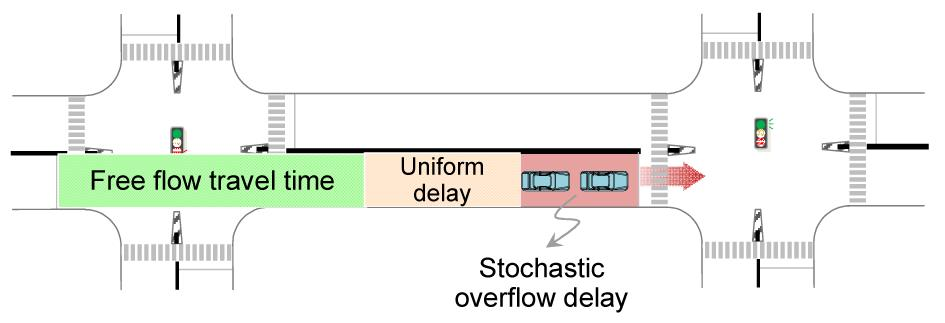Fig.1
Phenomenon of stochastic delays at signalized intersections

However, it is challenging to carry out such analysis in the case of urban arterials, given the interrupted nature of signals. The state-of-the-art methods mainly rely on empirical approaches, which have little physical meaning for explaining the fundamental mechanisms of travel times on urban arterials, and thus have limited transferability to different traffic conditions at different sites. This study aims to develop an analytical model to investigate delay variability at isolated, fixed-time controlled intersections, considering various influencing factors such as the degree of saturation, the evaluation period and the arrival distribution.

## 2.  Related works

To date, research on urban arterial travel time estimation has been quite limited because the interrupted nature of traffic flow on urban arterials makes such estimations more challenging than on freeways. However, such difficulties have led to an active interest in this topic, especially in recent years. In order to provide a foundation for this research, a review of literature focused on the stochastic delay model, delay variance models, and delay distribution models.

1. Stochastic delay model

Newell () explains that the expected delay per vehicle under a uniform and stochastic arrival pattern in nearly saturated (but not saturated) conditions consists of three parts: the deterministic delay, the stochastic delay under saturated conditions, and the delay to vehicles if they cannot pass through the intersection in one cycle, which is also stochastic. The deterministic delay has been discussed in classic works by Webster (). The second type of delay is much smaller than the other delays. The third component is the delay caused by stochastic overflow.

2. Delay variance models

Fu and Hellinga () developed a delay variability model to quantify the variation in delays in highly undersaturated and highly oversaturated conditions. Gu and Lan () questioned whether the single empirical curve in Fu and Hellinga ()’s model fits the variability well during traffic conditions between highly undersaturated and highly oversaturated, and therefore developed an alternative model based on statistical distributions of traffic arrivals at different degrees of saturation to predict delay variability. The model contains two components, the expected conditional variance of the individual delay and the variance of the mean delay. For practical applications, the expression is further simplified using the Taylor expansion. Finally, a Monte Carlo simulation is conducted to verify the proposed variability model. It is indicated that Fu and Hellinga ()’s model seems only to contain the expectation of the conditional variance and tends to underestimate the overall delay variability over mid to high degrees of saturation.

3. Delay distribution models

Another way to model delay variability is to derive its probability distribution. Due to stochastic arrival and departure processes, the operated delay at an intersection could be significantly different from that expected. In this case, a range of delay values with a particular probability distribution will seem more appropriate in practice. However, limited research can be found on this topic. Olszewski () developed an average delay (per cycle) probability distribution model based on a sequential calculation of the queue length probability. He explicitly investigated the delay distribution under the influence of arrival distributions, the degree of saturation, and various control parameters. Zheng () proposed an analytical method for the estimation of the urban link delay distribution. The results indicate a correlation between arrival time and link travel time under different degrees of congestion. The models also demonstrate the evolution of the delay distributions. Both the average and the variance of the delay increase cycle by cycle. However, the simplification of the signal control, to have the same cycle time and green splits at neighboring intersections, limits its application to real-life situations. Besides, the overflow queues at continuous intersections along arterials have not been fully investigated. How to efficiently utilize delay distribution methods for arterial performance evaluation in decision-making processes thus remains a critical issue.

Considering the above, the stochastic nature of arterial traffic flow under signal controls has not been fully interpreted by deterministic delay models. A stochastic modeling approach is urgently needed to reflect the delay uncertainty and delay distribution for arterial travel time estimation. Accordingly, an analytical model is proposed in this study to investigate delay variability at isolated, fixed-time controlled intersections. The interpretation of delay evolvement at intersections will give a comprehensive insight into arterial travel time variability and provide further possibilities for travel time estimation.

## 3.  Delay variability modeling for isolated signalized intersections

### 3.1.  Delay components

To date, various analytical delay models have been developed to estimate the average delay under certain assumptions, e.g., a Poisson process and a constant arrival rate (Akcelik and Rouphail, ). Point estimates of the average delays are provided over the period of analysis (typically 15 min, 30 min or 1 h).

Take the delay estimation model in the HCM () for example. Three terms are involved in total: the uniform, random and initial queue delays. The uniform delay is related to the average waiting time of a vehicle approaching the intersection when the signal is red and no queue is present at the intersection. The random and initial queue delays are related to a range of volume-to-capacity ratios around unity. In some cycles, when traffic demand exceeds the approach capacity available for departures, a queue of vehicles will remain at the end of the green time in the current cycle, or the beginning of the green time in the next cycle. This phenomenon occurs either due to random fluctuations in the arrival flow rate (Fig. 2a), or during prolonged periods of oversaturation (Fig. 2b).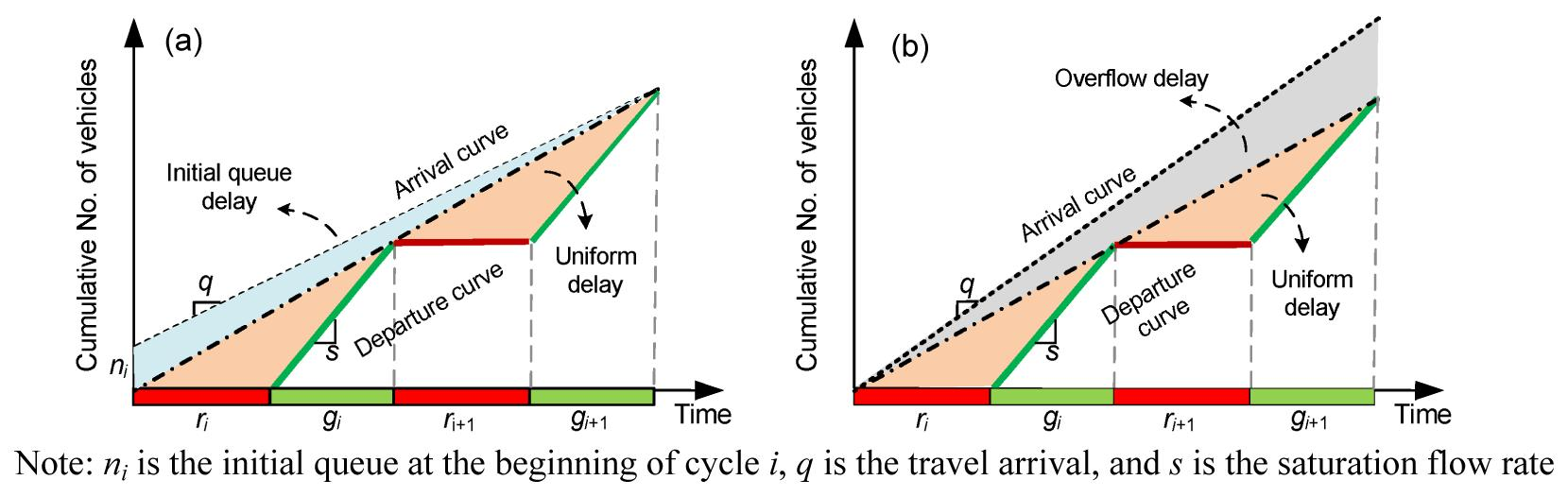Fig.2
Overflow delay illustration by cumulative arrival/departure curves
(a) Initial queue delay; (b) Random overflow delay

Abdy and Hellinga () proved that the randomness of vehicle arrivals results in a delay function that tends to be a uniform delay model at low v/c ratios and a deterministic oversaturation delay model at high v/c ratios. For v/c ratios in the range of 0.9 to 1.1, a non-linear relationship exists between the delay and the v/c ratio. The stochastic nature of traffic arrivals results in significantly higher delays than that estimated by standard deterministic queuing models. This causes the delay associated with random arrivals to be higher than the delay associated with uniform arrivals. Therefore, both uniform delay and overflow delay are focused on in this study when estimating the average delay per cycle.

### 3.2.  Definition of average delay per cycle

If no queue exists at the beginning (defined as the start of red) or end of the cycle, then the average delay can be calculated by the classical uniform delay model (Webster, ). Under overflow conditions, the precise definition of the average delay per vehicle per cycle becomes important. Conventionally, it is defined as the total delay incurred during period T (e.g., one cycle, 15 min) by all the vehicles arriving during cycle i, as shown in Fig. 3a, D 1, divided by the number of arrivals in cycle i. However, such an “average” does not reflect the true delay experienced by vehicles arriving during cycle i as the delay caused by the initial queue, D 2, is mistakenly included.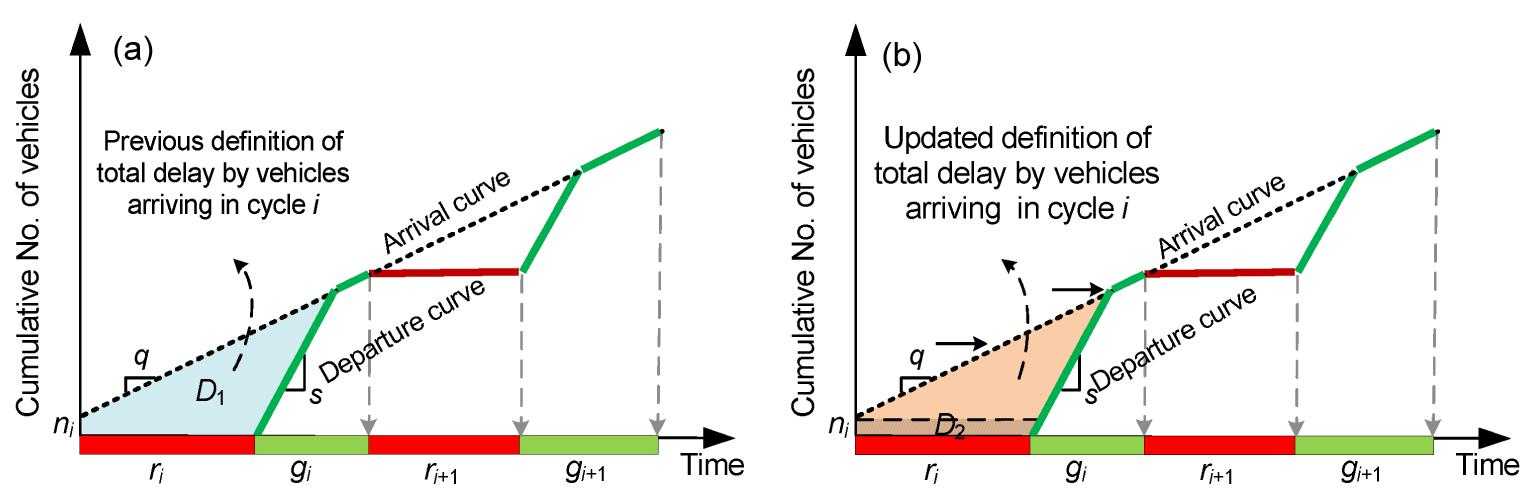Fig.3
Definition of average delay incurred per cycle
(a) Total delay in the cycle; (b) Delay for vehicles arriving during the cycle

The real delay for vehicles arriving during cycle i (in the undersaturation case) should be represented by the area D 1D 2, as shown by the orange area (in the web version) in Fig. 3b.

To compute the overflow delay experienced by each vehicle and also the average delay for the cycle, one needs to compute the time of this delay, from the arrival of the vehicle at the intersection until the moment it leaves the intersection, which can be several cycles later.

An overflow queue can exist at the beginning of the cycle. In that case, part of the delay during cycle i is incurred by vehicles that arrived during cycle i−1 (or earlier). If not all vehicles are able to depart during cycle i, some will incur an extra delay waiting for the green phase of cycle i+1 (or subsequent cycles, as shown in Fig. 4).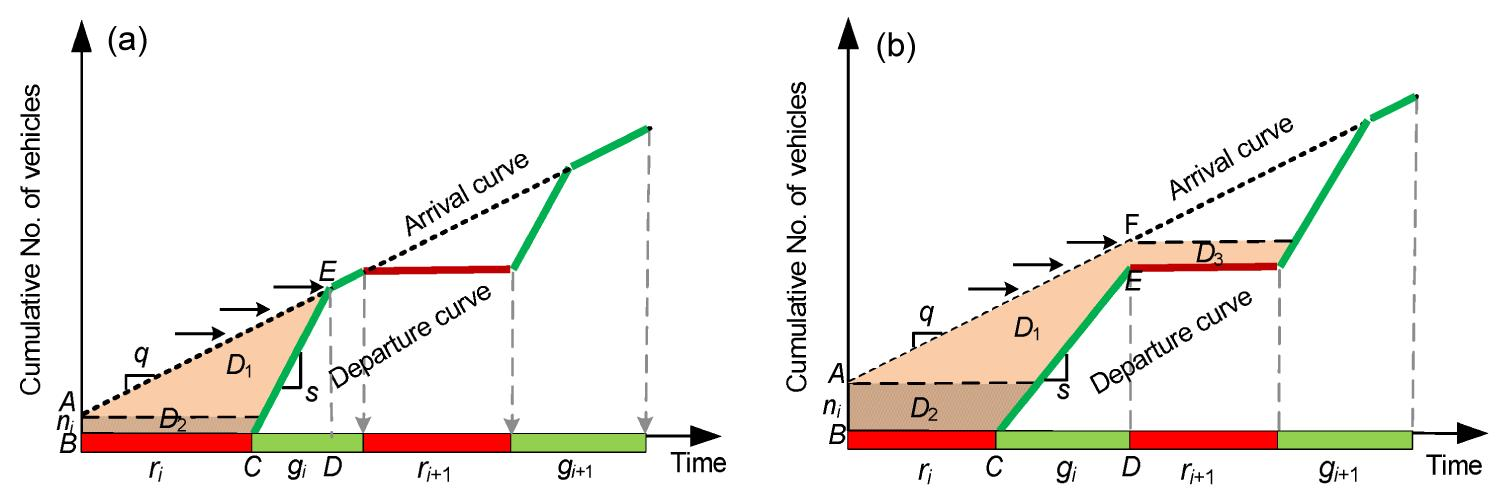Fig.4
Overflow queue at the beginning of the cycle (a) and overflow queue at both beginning and end of the cycle (b)

The general form of the overflow queue delay experienced by vehicles arriving during cycle i is represented by the areas as shown in Fig. 4: , where D 1 represents the total delay accumulated within cycle i by all vehicles arriving during cycle i and the initial queue ni at the beginning of cycle i; D 2 is the delay experienced only by vehicles in the initial queue ni at the beginning of cycle i; D 3 is the delay experienced by vehicles arriving during cycle i that is caused by the presence of the initial queue ni .

The following section will present a detailed derivation of the average delay across the cycle.

### 3.3.  Delay formulation with a deterministic initial queue

There follows a systematic computation of the average delay in one cycle based on the geometrical relationships introduced above. q represents the arrival rate (veh/s), t c stands for the cycle length, and s for the saturation flow rate (veh/s).

1. Area D 1

If ni +qt c<sg (undersaturation, Fig. 4a), , , , .

If ni +qt csg (oversaturation, Fig. 4b), , , .

To sum up, D 1 can be calculated as follows:

2. Area D 2

Note that it may take more than one cycle for the overflow queue ni to depart. Given saturation flow rate s, the overflow queue will decrease cycle by cycle according to the sequence ni , ni sg, ni −2sg, …, ni ksg, where , where the floor operator ⌊ ⌋ is used to indicate the integer value of the expression inside the brackets.

The area D 2 in Fig. 5a can be further divided into delay D 2r , which is incurred during the red periods, and D 2g , which is the delay incurred during the green periods. Thus, . D 2g can be calculated as .

D 2r is the sum of several rectangular areas over the red periods. So D 2 can be written as , where ni is the overflow queue at the beginning of cycle i, and k is the integer value of the ratio of ni to sg.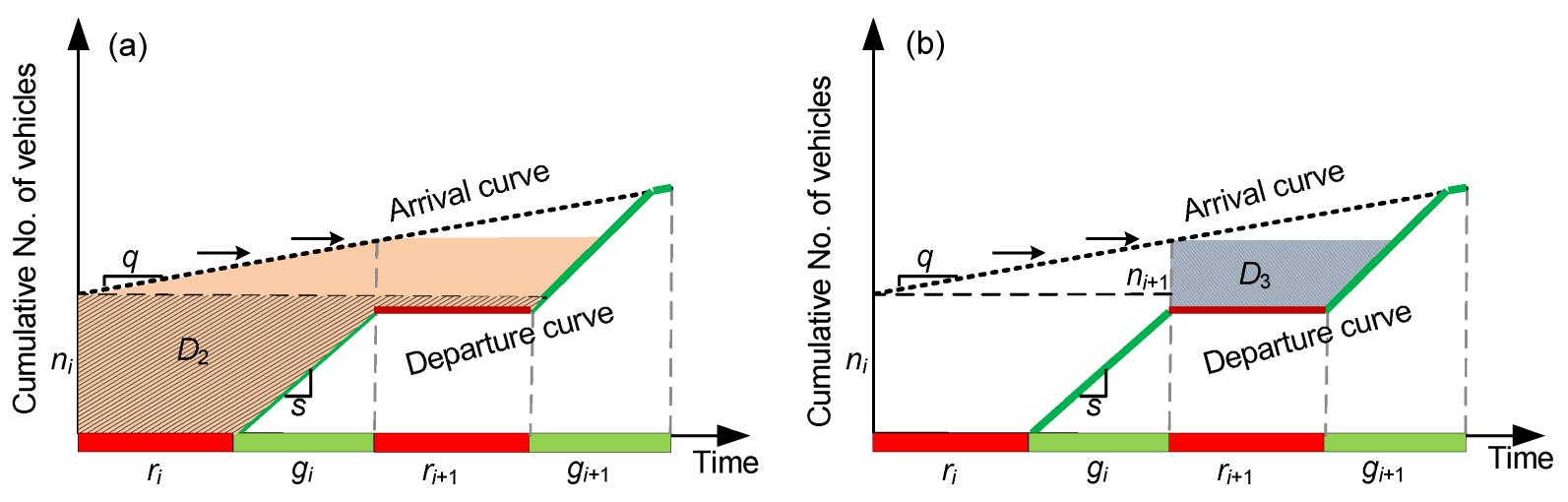Fig.5
Relationship between areas D 2 (a) and D 3 (b)

3. Area D 3

Eq. (13) can also be used to calculate area D 3 by replacing ni with ni +1, as illustrated in Fig. 5b: , .

Now, the average delay di for vehicles arriving during cycle i can be calculated by .

Taking the above equations together, in each cycle, the distribution of the delay only depends on the distribution of the overflow queues at the beginning of cycle i and the distribution of the arrivals during cycle i. Since these two characteristics have been assumed to be independent of each other, the probability of incurring a particular delay is computed by a simple multiplication of these two probabilities.

### 3.4.  Delay formulation with a stochastic initial queue

The delay probability distribution function derived above is based on a deterministic initial queue at the start of the green phase. If the initial queue is stochastic with a certain probability distribution, the expected probability distribution of the delay can be described as a weighted sum of probability functions (Zheng, ): , where P(ni ) is the probability distribution of the initial queue.

It can be derived using the Markov chain process by assuming a certain arrival distribution or departure distribution (Olszewski, ; Viti, ; van Zuylen and Viti, ). A detailed introduction to Markov Chain processes will be presented below.

The delay formulas above indicate that the delay distribution in each cycle only depends on the distribution of the queues at the beginning and end of the cycle and the distribution of arrivals during the cycle. Such a process can be illustrated using the analogy of Markov chains on a cycle-by-cycle basis. As shown in previous studies (Brilon and Wu, ; Olszewski, ; ; Viti, ), the Markov chain model enables one to consider the effects of stochastic arrival and service processes in the context of queues and delays.

A Markov chain is a random process whose state changes over time. A Markov chain is entirely defined by the current state and the transition probabilities. The transition probabilities are associated with the manner of state progression as the process evolves. A process with the Markov property satisfies the following: the conditional probability of the process being in a particular state at the next time point, given the current state, depends only on the current state and not on previous states of the system. Mathematically, this can be expressed as .

The Markov property captures the probabilistic nature of travel time. Traffic progresses like a Markov chain at intersections along urban arterials. The process by which traffic arrives and departs at signalized intersections has a stochastic nature; in particular, the initial queue length is the result of such a stochastic process. Fig. 6 illustrates both the input demand from upstream intersections and the service mechanism, i.e., the number of vehicles that pass through the intersection within one cycle.Fig.6
Illustration of Markov chain process at signalized intersections

The analytical representation of the system is given in Eq. (15). Unlike in the deterministic case, both the arrival and departure components of this formula can be stochastic values given by probability distributions. Therefore, the initial queue length at the beginning of each cycle also follows a particular distribution.

Note that the signalized intersection system is governed by a cyclic mechanism (Viti, ). This allows one to use discrete time steps, equal to the cycle length, and to calculate the initial queue length distribution at the beginning of every green period. Regardless of whether one is studying fixed-time controls with invariant cycle length or time-dependent controls with variable signal parameters, a state ni +1 is only dependent on the previous state ni and the number of arrivals and departures during the time interval, e.g., the cycle length. This method is also referred to as renewal theory (Markov, ).

### 3.5.  Basic assumptions

Considering the analogous properties of the Markov chain and the queuing process at signalized intersections, the dynamics of the initial queue length and its impact on the delay can be analyzed based on the probability distributions of the input demand and the service rate per cycle.

Regarding input demand, Dunne () derived expressions of delays at fixed-time signals and traffic-actuated controls by assuming a binomial arrival process. In addition, the arrivals at an isolated intersection are commonly assumed to follow a Poisson process. For example, van Zuylen () first described a Markov model for queues at isolated intersections assuming Poisson arrivals and normally distributed saturation flow rates. Later, Olszewski (; ) adopted the Poisson arrival process to model queue length evolvement over time at isolated intersections.

In real life the arrivals are usually different on a cycle-by-cycle basis, as shown in Fig. 7. A common way of dealing with this is to assume a stationary condition with a certain arrival distribution throughout the evaluation period, in which the average arrival rate does not change significantly. In Fig. 7, an average arrival rate of q has been assumed for all cycles within the evaluation period. In the case of a Poisson arrival distribution, this average value will define its shape and probability distribution, and determine the expected initial queue length in each cycle. Of course the Markov chain can be modeled using any distribution function. In practice, the distribution function can be determined by collecting a sufficient number of traffic counts under the same prevailing conditions and finding the most appropriate function to fit these observations.Fig.7
Illustration of arrival process at isolated intersections

The service rate means the maximum number of vehicles that can be dealt with by the intersection within one cycle. It is also regarded as the saturation flow rate, as shown in Chen et al. (). Previous studies have usually assumed the saturation flow rate to be a constant value (HCM, ; ) or to have a binomial (Olszewski, ; ) or normal (van Zuylen, ) distribution. In this study, saturation flow rate of the through lane is considered to be a constant value, independent of both queue length and arrival flow rate.

Besides the arrivals and departures in each cycle, the computation of queue length evolvement requires the specification of an initial queue length at the beginning of the evaluation period. This value can be obtained by comparing the traffic conditions to the previous period, as indicated in Eq. (15), or simply by observing the real queue length in the current cycle. Within the evaluation period, every cycle starts with a certain initial queue given the assumed arrival distributions.

The following is a brief summary of the assumptions that have been made:

1. The number of vehicle arrivals per cycle is a random variable with a known probability distribution. In the case of an isolated intersection, the Poisson distribution is employed.

2. Vehicle arrivals are uniformly distributed within each cycle.

3. The saturation flow rate of the through lane and the signal control parameters, i.e., green time and cycle length, are assumed to be constant.

4. The average delay across a cycle per arriving vehicle during a certain evaluation period is used as a measure of intersection performance. Variations in the delay incurred by individual vehicles arriving in the same cycle are not considered.

## 4.  Numerical case study of delay variability analysis

A numerical case study will be presented. Various factors are taken into account to investigate their effects on delay variability at isolated intersections.

### 4.1.  Evolvement of delay probabilities for different degrees of saturation

Given the basic scenario (green time g=24 s, cycle length t c=60 s, saturation flow rate s=1800 veh/h and a Poisson arrival distribution), an evaluation period of 30 min is set, with zero initial queue at the very beginning of the cycle. Typical degrees of saturation of 0.9, 1, and 1.1 are chosen to analyze the undersaturation, saturation and oversaturation cases, respectively.

Fig. 8a illustrates the evolution of the average delay within one cycle for the undersaturation case (x=0.9). It can be identified that the shape of the delay distribution changes very little after the first five cycles. Fig. 8b helps further show that the distribution of the average delay gradually approaches an equilibrium, as reflected by the continuous curves for the 10th to the 30th probability densities.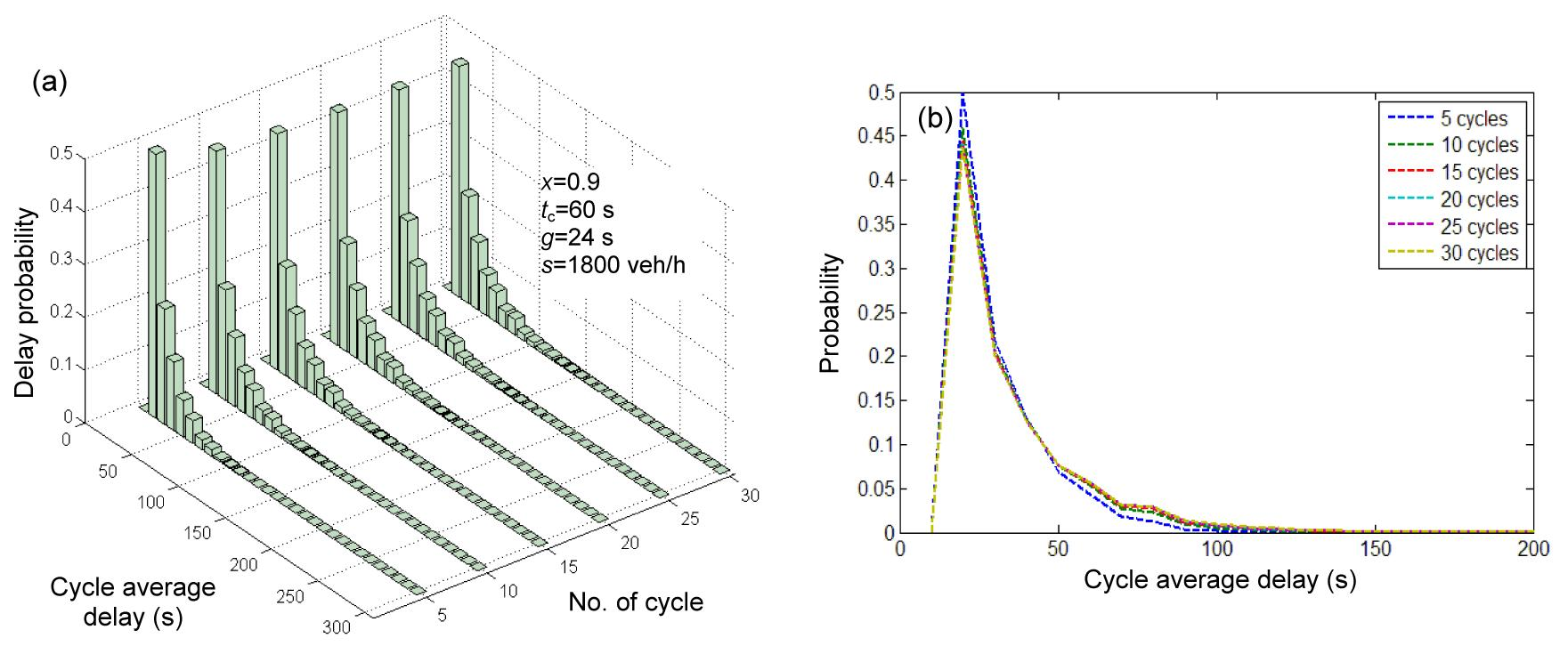Fig.8
3D view (a) and 2D view (b) of delay variability for x=0.9

In the full saturation case (x=1), as shown in Fig. 9, the shape of the delay distribution is time-dependent and becomes flatter with an increasing number of cycles. It is easy to conclude that later arrivals in a cycle are prone to being influenced by overflow queues and will thus incur longer delays, corresponding to uncertain traffic conditions.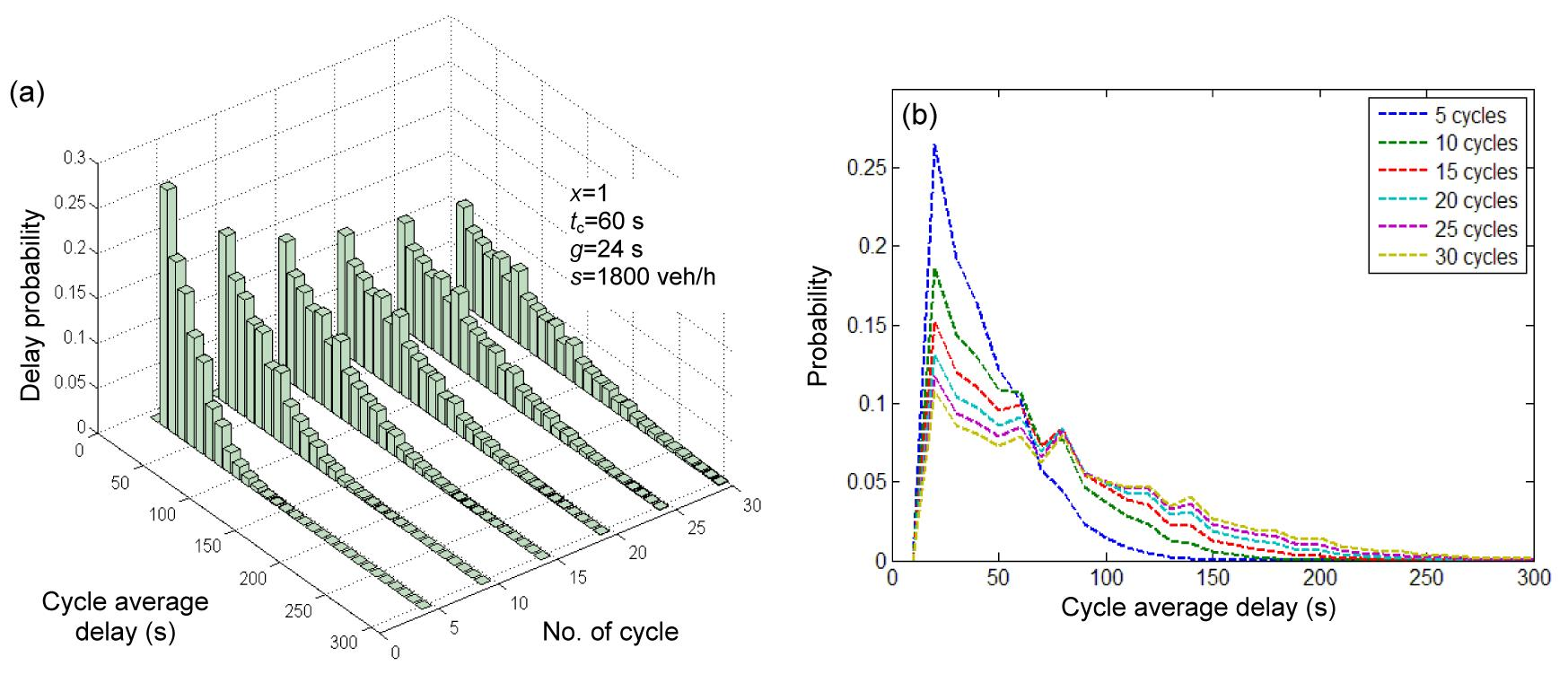Fig.9
3D view (a) and 2D view (b) of delay variability for x=1

In the oversaturation case (x=1.1), it has become deterministic that capacity cannot meet traffic demand. The variation in the delay distribution, or the probability of the overflow queue influencing subsequent cycles, increases rapidly over time (Fig. 10a). Compared to the full saturation case (x=1), the shape of the delay distribution shifts much more quickly to the right, with a high standard deviation. In addition, the delay distributions are closely overlapping (Fig. 10b), for which a group of level of service (LOS) can be characterized based on the average delay estimates.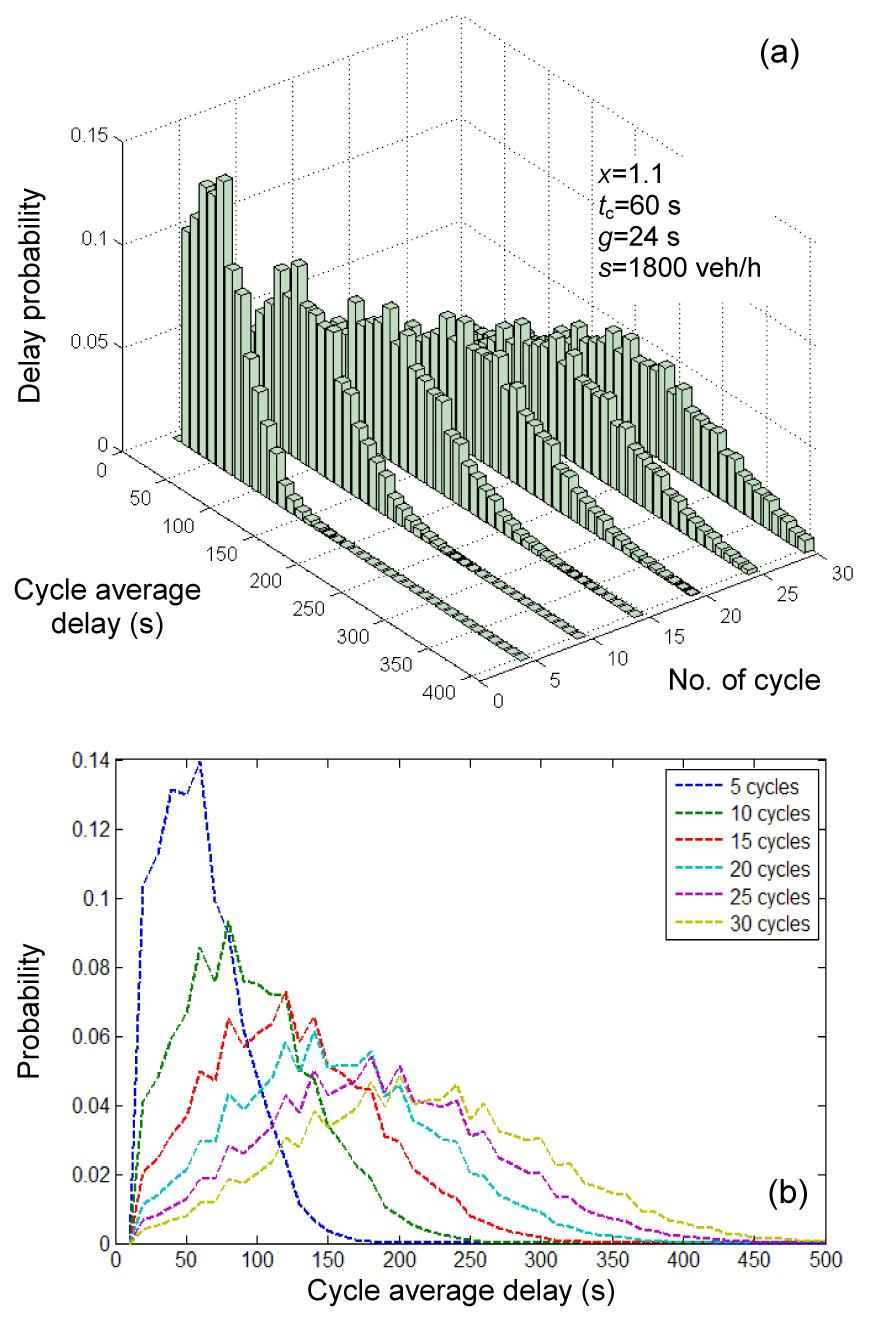Fig.10
3D view (a) and 2D view (b) of delay variability for x=1.1

The results confirmed the findings of Viti () and van Zuylen and Viti (). Meanwhile, they help strengthen the point that single delay estimation cannot give a full insight into the traffic state at an intersection.

### 4.2.  Evolvement of delay probabilities for different degrees of saturation

The analysis in Section 4.1 shows how the delay variability changes with the degree of saturation and with an increasing number of cycles. In practice, the values of interest are delay variability-related measures within the evaluation period, e.g., average delay, standard deviation, and confidence intervals.

Therefore, Olszewski () proposed averaging all of the cycle-by-cycle delay probabilities within the evaluation period. , where K is the number of cycles within the evaluation period and qij (k) represents the probability of realization of the state (Ak =i, nk =j) in cycle k. Ak =i is the number of arrivals in the cycle following a certain distribution, e.g., Poisson, and nk =j is the initial queue in that cycle also having a certain probability dependent on traffic arrival and departure in the previous cycle.

Eq. (19) indicates that the delay dk can be observed to have a certain distribution in a randomly selected cycle during the evaluation period. By assuming that the cycle-based traffic process is being repeated with the same flow rate and signal control throughout the evaluation period, the ‘averaged’ probability distribution represents the uncertainty for drivers who may arrive at the intersection at any time during the evaluation period.

According to HCM (), the time interval considered for performance evaluation is typically in the range of 15 min to 1 h, with longer durations within this range sometimes used for planning analyses. In general, caution should be taken when interpreting the results from an analysis period of 1 h or more because the adverse impact of short peaks in traffic demand may not be detected. Then, two commonly recommended time intervals for delay evaluation, 15 min and 30 min, are utilized in an average delay probability analysis.

Figs. 11a and 11b show the averaged delay distributions for T=15 min and 30 min, respectively. It is easy to identify that the shapes of the delay distributions for x=0.9 in both periods barely change. On the other hand, the delay distributions for x=1 and x=1.1 in the evaluation period of 30 min shift to the right, with higher values than for the 15-min period. Of the two saturation conditions, the case of x=1.1 apparently has the largest shifting distance and spread, with a wider range of delay. Similar to the tendency of the cycle-by-cycle delay distribution in the previous section, the average delay variability within a certain period is not sensitive to the time span in undersaturated conditions but is sensitive and time-dependent in saturated and oversaturated conditions.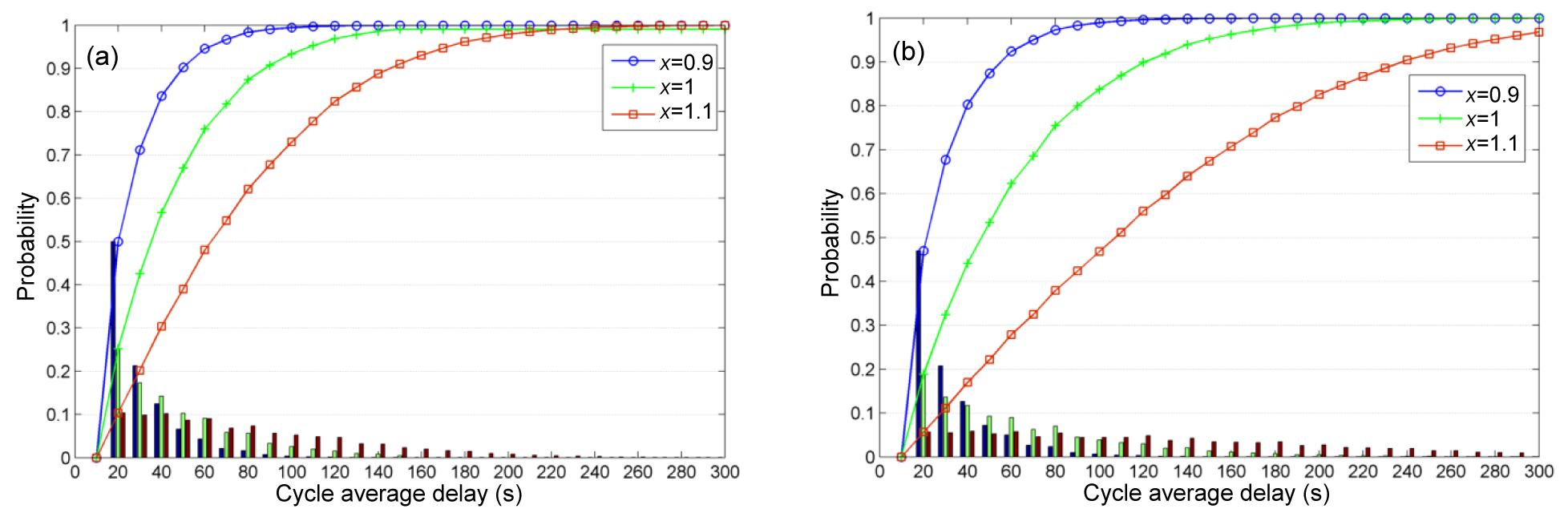Fig.11
Averaged delay distribution for T=15 min (a) and T=30 min (b)

For a more detailed comparison, the values of the mean delay, its standard deviation and the 90% confidence interval are calculated for degrees of saturation ranging from 0.7 and 1.2, for T=15 min and 30 min. The results are shown in Table 1. It can be seen that the mean values and standard deviations become larger and the confidence intervals or ranges of the delay estimates become wider with an increasing degree of saturation. However, the coefficient of variation is not monotonically increasing. After passing the full saturation condition of x=1, it starts to decrease, which implies the relatively deterministic nature of the delay in oversaturated conditions.

#### Table 1

Comparison of delay variability estimation for T=15 min and 30 min
 Evaluation period Degree of saturation Average delay per cycle estimates Mean±SD (s) Coefficient of variation 90% confidence interval Lower 5% (s) Upper 5% (s) T=15 min 0.7 16.29±4.64 0.28 12.46 25.14 0.8 19.47±8.56 0.44 12.96 36.80 0.9 27.06±16.74 0.62 13.88 61.71 1.0 44.56±31.11 0.70 14.73 108.00 1.1 74.66±49.89 0.67 17.05 171.64 1.2 113.26±70.85 0.63 21.77 243.53 T=30 min 0.7 16.32±4.70 0.29 12.46 25.14 0.8 19.68±8.91 0.45 12.96 37.71 0.9 29.03±19.33 0.67 14.09 69.46 1.0 59.00±44.35 0.75 15.43 148.20 1.1 122.06±81.98 0.67 18.38 278.86 1.2 198.39±121.25 0.61 29.71 472.80

With regard to the difference between T=15 min and 30 min, as already discussed, the delay distribution is more sensitive to the time span in the saturation and oversaturation cases compared to the undersaturation case. All the evaluation indices for the two evaluation periods are quite close to each other when the degree of saturation is less than unity. However, when the degree of saturation passes unity, a significant discrepancy occurs. For instance, the mean delay for x=1 and T=15 min is 44.56 s, compared to 59 s when T=30 min. Moreover, the difference becomes even more extreme when x=1.1 and 1.2. All these findings help to emphasize the importance of setting an appropriate evaluation period for delay variability analysis.

### 4.3.  Comparison with classic delay models

In order to evaluate the consistency of the average delay estimates produced by the proposed model compared to various classic models, e.g., the HCM (), ARRB (Akcelik, ) and Webster (), delay calculations are performed over the entire range of degrees of saturation under the following scenario: green time g=20 s, cycle length t c=60 s, saturation flow rate s=1800 veh/h, and a Poisson arrival distribution. The evaluation period is set as 30 min. Fig. 12 shows the results of the comparison and detailed estimates are presented in Table 2. For a better understanding of the impact of delay estimates, the level of service (LOS) criteria, based on control delay per vehicle (HCM, ; ), are also illustrated in Fig. 12.

#### Table 2

Average delay estimates produced by classic models
 Degree of saturation Uniform delay (s) Mean average delay per cycle estimates (s) Webster (1958) HCM (2000) ARRB (Akcelik, 1981) Proposed 0.1 11.25 11.52 11.52 11.25 11.01 0.2 11.74 12.33 12.36 11.73 11.53 0.3 12.27 13.21 13.34 12.27 12.05 0.4 12.86 14.17 14.52 12.86 12.88 0.5 13.50 15.26 15.99 13.50 13.69 0.6 14.21 16.61 17.92 14.21 14.70 0.7 15.00 18.57 20.71 15.25 16.32 0.8 15.88 22.35 25.38 19.92 19.68 0.9 16.88 34.14 35.51 30.55 29.03 1.0 18.00 – 65.43 63.74 59.00 1.1 18.00 – 130.08 133.51 122.06 1.2 18.00 – 211.92 218.21 198.39

First of all, it can be noted that there is a general agreement between the proposed model and the other three analytical models for lower degrees of saturation, e.g., x<0.5. This indicates that the randomness of vehicle arrivals can be neglected for highly undersaturated conditions, and the analytical models, whether deterministic or stochastic, can provide satisfactory results. However, discrepancies start to occur with increasing degrees of saturation. For example, at a degree of saturation of 0.7, the HCM gives delay estimates of over 20 s, corresponding to LOS C, whereas the estimates of the other three models correspond to LOS B. This reveals that the estimation of stochastic vehicle delay plays an importance role in accurately determining operational LOS at signalized intersections.

When the degree of saturation gets closer to unity, the delays given by the Webster () model tend to approach infinity, which is a well-known characteristic of that model. Besides, a larger difference is found between the HCM () model and the proposed model. This is due to the ‘algebraic manipulation’ in the coordinate transformation technique applied to realize the transition between undersaturated to oversaturated conditions (Dion et al., ). For the oversaturated cases, the estimates produced by the HCM (), ARRB (Akcelik, ), and the proposed model are in general agreement. Among them, ARRB (Akcelik, ) shows the best consistency, although a slight difference exists, as shown in Table 2. In general, the estimates produced by the classic delay models increase monotonically with the degree of saturation, and the estimates produced by the proposed model are consistent with these time-dependent delay models.

### 4.4.  Delay variability under different arrival distributions

In the analysis above, the arrival distribution is assumed to be a Poisson process. This may describe very well the traffic arrivals at isolated intersections with light traffic volumes. However, when traffic demand increases, vehicles start to form clusters or platoons with more uniform headway and less variance. In this case, the Poisson arrival distribution may not be appropriate. Other arrival distributions, e.g., the truncated Poisson, or the binomial (Olszewski, ; Viti, ) would probably be more applicable. Here, as an example, the binomial arrival distribution is utilized to assess how different arrival distributions influence estimated delay variability under different traffic conditions.

Compared to the Poisson distribution with equal mean and variance, the ratio of variance to mean (hereafter referred to as the I ratio) is less than 1 for the binomial distribution. Of course, in practice, the I ratio needs to be determined based on real observations. Here, this ratio is set as 0.4, 0.6 and 0.8, for illustration purposes. The results are compared with those for the Poisson process, for both undersaturated and oversaturated conditions (Fig. 13).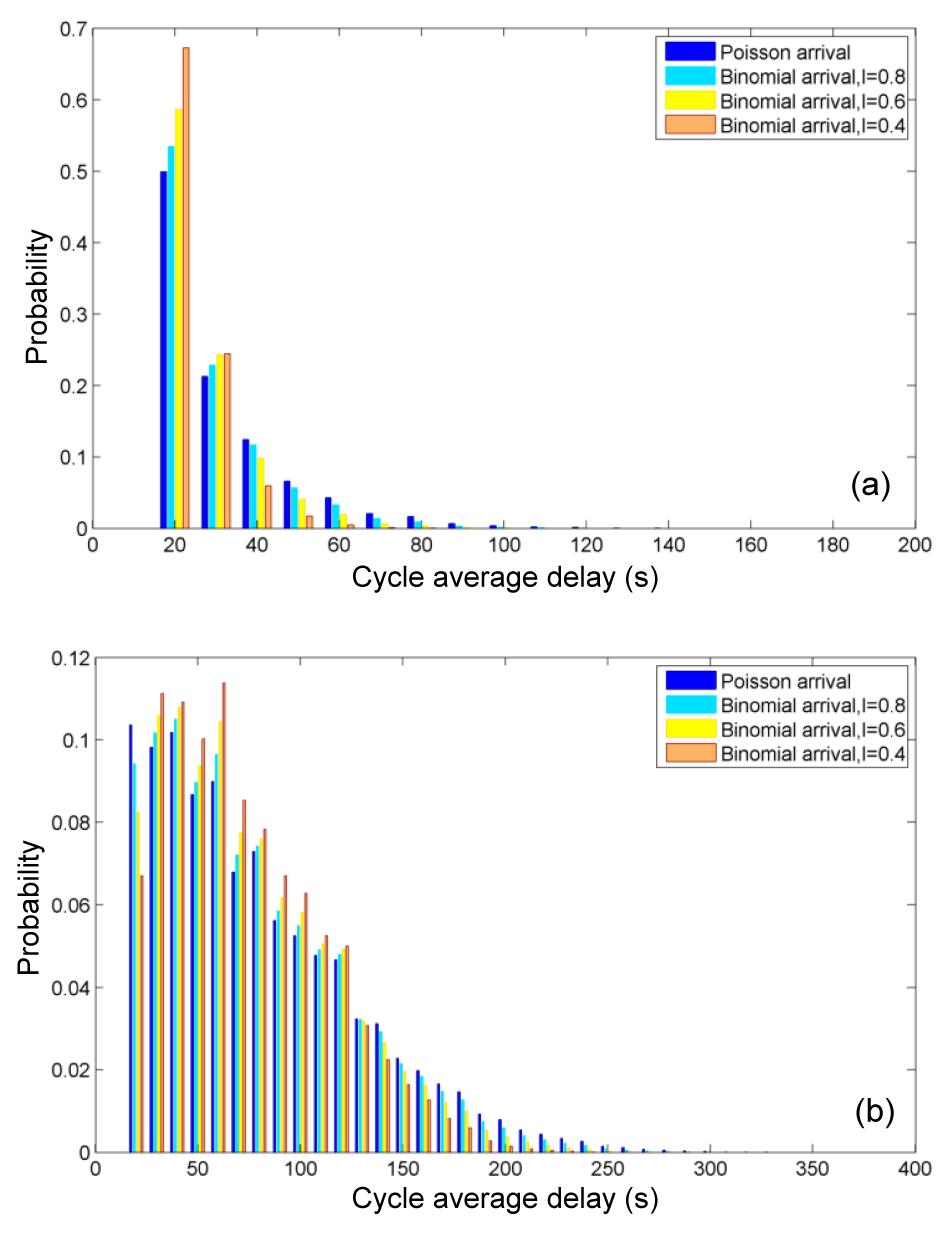Fig.13
Delay distributions under Poisson and binomial arrival distributions
(a) Undersaturated conditions, x=0.9, T=15 min; (b) Oversaturated conditions, x=1.1, T=15 min

Under both conditions, the delay estimates produced by binomial arrival distributions with larger I ratios tend to have a wider distribution. Apparently, a larger range of delays are covered by the estimation in oversaturated conditions. This is reasonable because, in the case of a Poisson arrival process with I=1, the arrival flow is more uncertain, leading to a larger variance in the delays experienced by vehicles at the intersection. On the other hand, a binomial arrival distribution with a lower I ratio represents less variance in the arrival flow, so that vehicles travel in clusters and utilize the green time more efficiently. Correspondingly, a narrower distribution of delays can be expected in this case.

This indicates that the variability of arrivals has a significant effect on the delay estimates. A larger variance in the arrival flow will result in a larger variance in the delay distribution. In practice, more analyses are needed on the types of arrival distributions corresponding to different traffic conditions. In this regard, along urban arterials, the signal control at an upstream intersection strongly impacts the arrival flow at a downstream intersection. The relationships between signal coordination and arrival distribution, and further the delay estimation, require additional attention in future studies.

## 5.  Conclusions and future work

Travel time variability on urban arterials is largely dependent on the delay variability vehicles experience at signalized intersections. The interpretation of delay evolvement at intersections can give comprehensive insights into arterial travel time variability and provide further possibilities for travel time estimation. Therefore, an analytical model was proposed in this paper to investigate average cycle delay variability at isolated, fixed-time controlled intersections whereas in most research only point estimates of delay were provided.

The delay formulas were firstly derived using cumulative curves, assuming a deterministic initial queue; later on, the stochastic nature of the overflow queue was investigated using the analogy of a Markov chain process. Then, a numerical case study of delay variability analysis was conducted, taking into account various factors. The main findings are summarized below:

1. It has been confirmed that different degrees of saturation lead to different shapes of delay distribution. In undersaturated conditions, the shape of the delay distribution changes very little over time. In saturated and oversaturated conditions, however, the delay distributions are time-dependent and become flatter with an increasing number of cycles.

2. Delay variability was averaged over the cycles within the evaluation period to provide variability-related measures of interest, e.g., average delay, standard deviation, and confidence intervals. Two time spans, 15 min and 30 min, were investigated. It was found that the delay distribution is more sensitive to the time span in saturated and oversaturated than in undersaturated conditions. All of the evaluation indices for the two evaluation periods are quite close when the degree of saturation is less than unity. However, a significant discrepancy occurs when the degree of saturation goes beyond unity.

3. The average delay estimates produced by the proposed model were compared to those from various classic models (HCM (), ARRB (Akcelik, ) and Webster ()) over a wide range of degrees of saturation from 0.1 to 1.2. In general, it was found that the estimates produced by the proposed model are consistent with these time-dependent delay models.

4. In order to assess how different arrival distributions influence delay variability in different traffic conditions, the binomial arrival distribution was utilized for illustration purposes. It was concluded that the variability of arrivals has a significant effect on delay estimates, in both undersaturated and oversaturated conditions. A larger variance of arrival flow results in a larger variance of delay distribution.

In summary, the ability to capture the characteristics of delay variability serves as a critical step in estimating and predicting urban travel times. For arterial performance evaluation, gaining knowledge of delay variability makes it possible to estimate the confidence limits around the mean delays and thus provide a more accurate and informative comparison of alternative control plans. However, the delay distribution model is only developed for isolated intersections in this study. In practice, traffic flows on urban arterials are controlled by signal coordination plans across multiple intersections. Delay or travel time is thus influenced by non-uniform arrivals, which can be easily reflected in the cumulative curves by assuming two different arrival rates at green and red phases. The assumptions of the same green time and cycle length made in previous work (Viti, ; Zheng, ) for consecutive intersections along arterials can also be relaxed to different signal plans. Additionally, the focus on identifying queuing delay patterns based on field data also facilitates the estimation of delay variability, as shown in (Ban et al., ; ). This will form the basis of our future work.

* Project supported by the National Natural Science Foundation of China (No. 51278455), the National Science Foundation for Post-doctoral Scientists of China (No. 2012M521175), and the Excellent Post-doctoral Science of Foundation of Zhejiang Province (No. Bsh1202056), China

## ReferencesAbdy, Z., Hellinga, B., 2008. Modeling day-to-day variability of intersection performance using microsimulation. Transportation Research Record: Journal of the Transportation Research Board, 2088:18-25.Akcelik, R., 1981.  Traffic Signals: Capacity and Timing Analysis. Research Report No. 123. Australian Road Research Board,Vermount South :Akcelik, R., Rouphail, N.M., 1994. Overflow queues and delays with random and platooned arrivals at signalized intersections. Journal of Advanced Transportation, 28(3):227-251.Ban, X.G., Herring, R., Bayen, A.M., 2009. Delay pattern estimation for signalized intersections using sampled travel times. Transportation Research Record: Journal of the Transportation Research Board, 2130:109-119.Ban, X.G., Hao, P., Sun, Z.B., 2011. Real time queue length estimation for signalized intersections using sample travel times from mobile sensors. Transportation Research Part C: Emerging Technologies, 19(6):1133-1156.Brilon, W., Wu, N., 1990. Delays at fix-time traffic signals under time-dependent traffic conditions. Traffic Engineering and Control, 31(12):623-631.Chen, P., Nakamura, H., Asano, M., 2011. Analysis of Saturation Flow Rate Fluctuation for Shared Left-turn Lane at Signalized Intersections in Japan: a Case Study. , TRB 90th Annual Meeting, Washington DC, :Dion, F., Rakha, H., Kang, Y.S., 2004. Comparison of delay estimates at under-saturated and over-saturated pre-timed signalized intersections. Transportation Research Part B: Methodological, 38(2):99-122.Dunne, M.C., 1967. Traffic delay at a signalized intersection with binomial arrivals. Transportation Science, 1(1):24-31.Fu, L., Hellinga, B., 2000. Delay variability at signalized intersections. Transportation Research Record: Journal of the Transportation Research Board, 1710:215-221.Gu, X., Lan, C., 2009. Estimation of Delay and Its Variability at Signalized Intersections. , TRB 88th Annual Meeting, Washington DC, :HCM (Highway Capacity Manual), 2000.  . Transportation Research Board,Washington DC :HCM (Highway Capacity Manual), 2010.  . Transportation Research Board,Washington DC :Jin, S., Huang, Z.Y., Tao, P.F., Wang, D.H., 2011. Car-following theory of steady-state traffic flow using time-to-collision. Journal of Zhejiang University-SCIENCE A (Applied Physics & Engineering), 12(8):645-654.Li, L., Shi, P., 2006. Numerical analysis on car-following traffic flow models with delay time. Journal of Zhejiang University-SCIENCE A, 7(2):204-209.Markov, A.A., 1971. Extension of the Limit Theorems of Probability Theory to a Sum of Variables Connected in a Chain.  Dynamic Probabilistic Systems.  John Wiley and Sons,New York :552-557.Newell, G.F., 1965. Approximation methods for queues with application to the fixed-cycle traffic light. SIAM Review, 7(2):223-240.Olszewski, P.S., 1990. Traffic signal delay model for non-uniform arrivals. Transportation Research Record, 1287:42-53.Olszewski, P.S., 1990. Modeling of Queue Probability Distribution at Traffic Signals.  . 11th International Symposium on Transportation and Traffic Theory, Tokyo, :Olszewski, P.S., 1994. Modeling probability distribution of delay at signalized intersections. Journal of Advanced Transportation, 28(3):253-274.Texas Transportation Institute and Cambridge Systematics, 2006.  Travel Time Reliability: Making It There on Time, All the Time. Federal Highway Administration,USA :van Zuylen, H.J., 1985. The Dynamics of Queues at Traffic Lights.  Dutch Lecture Notes VB41. Tilburg,Verkeersakademie :van Zuylen, H.J., Viti, F., 2007. A Probabilistic Model for Queues, Delays and Waiting Time at Controlled Intersections. , TRB 86th Annual Meeting, Washington DC, :Viti, F., 2006.  The Dynamics and the Uncertainty of Delays at Signals. PhD Thesis, Delft University of Technology,Delft, the Netherlands :Webster, F.V., 1958.  Traffic Signal Settings. Road Research Laboratory Technical Paper. Her Majesty Stationary Office,London :Zheng, F., van Zuylen, H.J., 2010. Uncertainty and predictability of urban link travel time: a delay distribution based analysis. Transportation Research Record: Journal of the Transportation Research Board, 2192:136-146.Zheng, F.F., 2011.  Modeling Urban Travel Time. PhD Thesis, Delft University of Technology,Delft, the Netherlands :

Open peer comments: Debate/Discuss/Question/Opinion

<1>

Please provide your name, email address and a commentJournal of Zhejiang University-SCIENCE, 38 Zheda Road, Hangzhou 310027, China
Tel: +86-571-87952783; E-mail: cjzhang@zju.edu.cn
Copyright © 2000 - Journal of Zhejiang University-SCIENCE